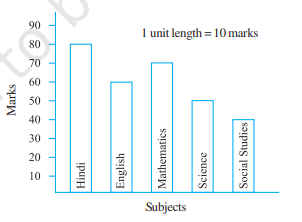# Observe this bar graph which shows the marks obtained by Aziz in half-yearly examination in different subjects.Answer the given questions.(a) What information does the bar graph give?(b) Name the subject in which Aziz scored maximum marks.(c) Name the subject in which he has scored minimum marks.(d) State the name of the subjects and marks obtained in each of them."

Given:

The bar graph shows the marks obtained by Aziz in half-yearly examination in different subjects.

To do:

Solution:

From the given bar graph, we can observe that

Marks obtained in Hindi $=80$

Marks obtained in English $=60$

Marks obtained in Mathematics $=70$

Marks obtained in Science $=50$

Marks obtained in Social Studies $=40$

Therefore,

(a) The bar graph shows the marks obtained by Aziz in different subjects.

(b) Marks obtained in Hindi $=80$

Therefore,

Aziz scored maximum marks in Hindi.

(c) Marks obtained in Social Studies $=40$

Therefore,

Aziz scored minimum marks in Social Studies.

(d) Hindi – 80 marks

English – 60 marks

Mathematics – 70 marks

Science – 50 marks

Social Studies – 40 marks